# Largest BST subtree in the given Binary Tree

## Introduction:

You are given a Binary Tree, and you need to return the size of the largest subtree of the given Binary Tree, which is a BST(Binary Search Tree). If, in any case, your entire Binary Tree is the BST, return the size of the whole Binary Tree.

Let us see a few examples:

INPUT:

OUTPUT: 3

EXPLANATION: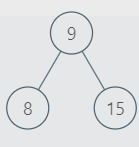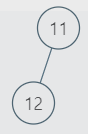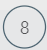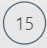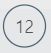These are the only SubTrees that are BSTs. So, the largest BST subtree among them has size 3. Hence, the output is 3.

INPUT:

OUTPUT: 6

EXPLANATION: Since the entire Binary Tree is the BST. Hence, the largest BST Subtree is of size 6.

## Approach 1:

You can run any(inorder, preorder, postorder) traversal on the tree and check if the subtree rooted at your current node is BST or not. In this way, you can find the largest BST subtree size.

Please refer to the below code for the approach mentioned above.

Time Complexity: The time complexity for the above code is O(N ^ 2) (where ‘N’ denotes the number of nodes in the given binary tree) because, for each node, we check if the subtree rooted at the current node is BST. We call this function for each node, and  ‘isBST()’ runs in O(N) time. Hence, the time complexity is O(N * N). Also time complexity of size() function is O(N).

Space Complexity: We are not using any auxiliary space, but we are using the recursive call stack space of O(N).

## Approach 2:

In approach 1, for each node, we checked its subtree for it to be a part of BST. But, what if we iterate from the bottom and return some information to the node to check if the current node subtree is BST or not in constant time(O(1)).

We will start from the leaf nodes, and the information which we would receive from its child would include the following things:-

1. If the child subtree is BST or not.
2. Maximum and Minimum value of all the nodes of child subtrees.
3. Size of child subtree if it is BST else -1.
4. Maximum answer till the child.

Now, how to check if the current node subtree is BST or not in O(1) time!

You can apply the following checks to check that:-

1. curNode.data > left.max (left.max: Maximum node value of left subtree)
2. curNode.data < right.min (right.min: Minimum node value of right subtree)

If the above two conditions satisfy, that means your current node subtree is a BST because a node’s value should be greater than the maximum value of its left child subtree and smaller than the minimum value of its right child subtree.

In this way, you can find the largest BST subtree size.

Please refer to the below code for the approach mentioned above.

Time complexity: The time complexity for the above code is O(N) because we only traverse the tree once.

Space Complexity: Constant auxiliary space is used. We are just using O(N) recursive call stack space.

## FAQ:

Approach1:

1. What is the time complexity of the isBST function?
The time complexity of the isBST function is O(N) because we run an inorder traversal inside this function whose time complexity is O(N).

2. What is the time complexity of the size function?
The time complexity of the size function is O(N) because we run an inorder traversal inside this function whose time complexity is O(N).

3. For what min and max are used in the isBST function?
The min and max specify the range in which the current node’s value should lie to follow the BST property.

Approach 2:

1. How did we come to the optimized approach for the problem of finding the Largest BST subtree?
Earlier in approach 1, we traversed from up to down and checked if its subtree is BST or not for each node. But in approach 2, we started from down to up and used information returned by the child to the parent to figure out if the current node subtree is BST or not.

2. How do we determine if the current node subtree is a BST or not?
The current node’s value should be greater than the left child’s subtree maximum value and smaller than the right child’s minimum value.

## Key Takeaways:

In this blog, we have covered the following things:

1. How to find the largest BST subtree size.
2. We optimized our approach 1 from O(N * N) approach to O(N) approach.

Until then, All the best for your future endeavors, and Keep Coding.